Ch 1. Particle General Motion Multimedia Engineering Dynamics Position,Vel & Accel. Accel. varyw/ Time Accel. Constant Rect. Coordinates Norm/Tang. Coordinates Polar Coordinates RelativeMotion
 Chapter - Particle - 1. General Motion 2. Force & Accel. 3. Energy 4. Momentum - Rigid Body - 5. General Motion 6. Force & Accel. 7. Energy 8. Momentum 9. 3-D Motion 10. Vibrations Appendix Basic Math Units Basic Equations Sections Search eBooks Dynamics Fluids Math Mechanics Statics Thermodynamics Author(s): Kurt Gramoll ©Kurt GramollDYNAMICS - EXAMPLE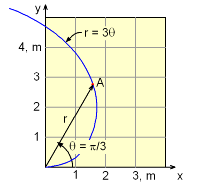Wire with Bead Example A curved wire is in the shape of r = 3θ. If a bead, point A, moves along the curve at a constant rate of dθ/dt = 8 rad/s, what is the acceleration magnitude at A? Distance units are meters. Solution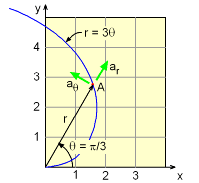Acceleration Vectors of Bead The total acceleration is a combination of both the r and θ accelerations.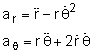The r terms can be found by taking derivatives,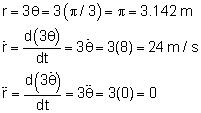The θ terms are given,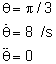Combining equations and terms give,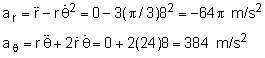The total acceleration is      a = (ar2 + aθ2)0.5 = ( -201.12 + 3842)0.5 =       v = 433.5 m/s2

Practice Homework and Test problems now available in the 'Eng Dynamics' mobile app
Includes over 400 problems with complete detailed solutions.
Available now at the Google Play Store and Apple App Store.